# Q-Learning

Q-learning [Watkins,1989] is a recent form of Reinforcement Learning algorithm that does not need a model of its environment and can be used on-line.

## The algorithm

Let the world state at timebe, and assume that the learning system then chooses action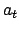. The immediate result is that a reward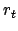is received by the learner and the world undergoes a transition to the next state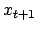. The objective of the learner is to choose actions maximizing discounted cumulative rewards over time. More precisely, letbe a specified discount factor in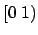. The total discounted return (or simply return) received by the learner starting at timeis given by: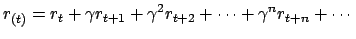(1)

The discount factoris a number in the range ofand is used to weight near term reinforcement more heavily than distant future reinforcement. The closeris to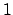the greater the weight of future reinforcements.

The objective is to find a policy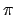, or rule for selecting actions, so that the expected value of the return is maximized. It is sufficient to restrict attention to policies that select actions based only on the current state (called stationary policies) :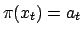. For any such policyand for any state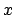we define: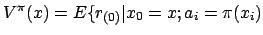for all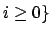(2)

The expected total discounted return received when starting in state x and following policythereafter. Ifis an optimal policy we also use the notation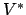for. Many dynamic programming-based reinforcement learning methods involve trying to estimate the state values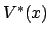orfor a fixed policy.

Q-learning, is a simple incremental algorithm developed from the theory of dynamic programming [Ross,1983] for delayed reinforcement learning. In Q-learning, policies and the value function are represented by a two-dimensional lookup table indexed by state-action pairs. Formally, for each stateand action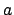let: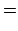(3)(4)

where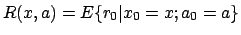andis the probability of reaching state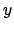as a result of taking actionin state. It follows that(5)

Intuitively, Equation 4 says that the state-action value,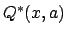, is the expected total discounted return resulting from taking actionin stateand continuing with the optimal policy thereafter. More generally, the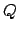function can be defined with respect to an arbitrary policyas(6)

andis justfor an optimal policy.

The Q-learning algorithm works by maintaining an estimate of thefunction, which we denote by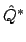, and adjustingvalues (often just called Q-values) based on actions taken and reward received. This is done using Sutton's prediction difference, or TD error [Sutton,1988] - the difference between (the immediate reward received plus the discounted value of the next state) and (the Q-value of the current state-action pair):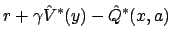(7)

whereis the immediate reward,is the next state resulting from taking actionin state, and. Then the values ofare adjusted according to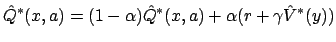(8)

where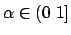is a learning rate parameter. Note that the current estimate of thefunction implicitly defines a greedy policy by. That is, the greedy policy is to select actions with the largest estimated Q-value.

It is important to note that the Q-learning method does not specify what actions the agent should take at each state as it updates its estimates. In fact, the agent may take whatever actions it pleases. This means that Q-learning allows arbitrary experimentation while at the same time preserving the current best estimate of states' values. This is possible because Q-learning constructs a value function on the state-action space, instead of the state space. It constructs a value function on the state space only indirectly. Furthermore, since this function is updated according to the ostensibly optimal choice of action at the following state, it does not matter what action is actually followed at that state. For this reason, the estimated returns in Q-learning are not contaminated by "experimental" actions [Watkins,1989], so Q-learning is not experimentation-sensitive.

To find the optimal Q function eventually, however, the agent must try out each action in every state many times. It has been shown [Watkins,1989 ; Dayan,1992] that if equation 8 is repeatedly applied to all state-action pairs in any order in which each state-action pair's Q-value is updated infinitely often, thenwill converge toand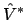will converge towith probability 1 as long as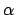is reduced to 0 at a suitable rate. This is why the policy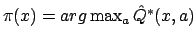is only used a part of the time in order to be able to explore the state-action space completely. At each iteration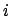, we will choose to make either a random action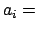random or the optimal action. We will take the first possibility (the random action) with a probability of. At the beginning of the learningmust be huge (near 1). At the end of the learning, when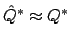, we will setto always use the optimal policy. It is, as always, the compromise between exploitation-exploration.

Shortly, we have:

•: learning rate
•: discount factor The closeris tothe greater the weight is given to future reinforcements.
•: probability to use a random action instead of the optimal policy.

## The robot Applet.

An illustration of these concepts is the robot example. At each time step, the robot can choose one of the following action:

• move its arm (yellow line) up.
• move its arm (yellow line) down.
• move its hand (red line) right.
• move its hand (red line) left.

The reward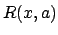the robot gets is simply the number of pixel on the screen it has moved (positive values for movement to the right and negative values for movement to the left). The goal is that the robot move to the right the faster possible

.

Some remarks:

• You can see on the upper-left part of the screen the average speed of the robot. That is:

 average speed(9)

When you press the [reset speed counter] button, the program set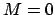and.

• Small value ofwill not allow the robot to extend its arm forward to the right because during all this time the robot gets no reward. You must set a high value forotherwise the robot doesn't see that, far in the future, it is useful to have its arm extended.

•should be set a high value (.8) at the beginning of the learning process.should be set to zero when the optimal control policy has been found. To see if an optimal policy has been found, press the [reset speed counter] button and wait a little until the [speed counter] stabilizes. You have now a correct evaluation of the speed of the robot. Continue the learning process until you can't increase this value anymore.

• You can compete with the algorithm! See by yourself that the Q-learning is the best! Try to beat it! How to play? Press the [stop] button. Press the [reset speed counter] button. Move the arm and the hand of the robot using the 4 arrow keys: [UP/DOWN] moves the arm (yellow line) and [LEFT/RIGHT] move the hand (red line). Move the robot until the [speed counter] stabilizes. Now the result: Do you really go faster than Q-learning?

## Bibliography

[Ross,1983] Ross, S. (1983). Introduction to Stochastic Dynamic

[Sutton,1988] Sutton, R. S. (1988). Learning to predict by the methods of temporal differences. Machine Learning 3:9-44.
[Watkins,1989] Watkins,C. (1989). Learning from Delayed Rewards,Thesis,University of Cambridge,England.
[Dayan,1992] Dayan, P. (1992). The convergence of TD(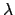) for general. Machine Learning 8:117-138.

## Download&links

The JAR-file of the applet (which can also be run as a stand-alone program) is here.
The source code of the applet is here (1 file).
The mathematical parts of this page are downloadable in PDF.

Reinforcement Learning Repository : you will find there an excellent illustrated tutorial which is mirrored here.
You can download A short Introdution to Reinforcement Learining by Stephan ten Hagen and Ben Kröse.
The on-line book by Sutton & Barto Book,"Reinforcement Learning: an introduction" is mirrored here.
A link to a Reinforcement Learning site